Fitting Linear Models > Statistical Details > Inverse Prediction with Confidence Limits
Publication date: 07/30/2020

# Inverse Prediction with Confidence Limits

Inverse prediction estimates a value of an independent variable from a response value. In bioassay problems, inverse prediction with confidence limits is especially useful. In JMP, you can request inverse prediction estimates for continuous and binary response models. If the response is continuous, you can request confidence limits for an individual response or an expected response.

The confidence limits are computed using Fieller’s theorem (1954), which is based on the following logic. The goal is predicting the value of a single regressor and its confidence limits given the values of the other regressors and the response.

Let b estimate the parameters β so that we have b distributed as N(β,V).

Let x be the regressor values of interest, with the ith value to be estimated.

Let y be the response value.

We desire a confidence region on the value of x[i] such that βx = y with all other values of x given.

The inverse prediction is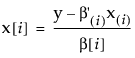where the subscript (i) in parentheses indicates that the ith component is omitted. A confidence interval can be formed from the relation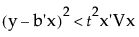where t is the t value for the specified confidence level.

The equation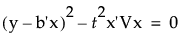can be written as a quadratic in terms of z = x[i]: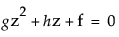where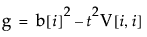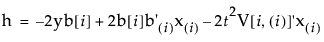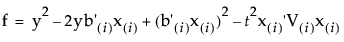Depending on the values of g, h, and f, the set of values satisfying the inequality, and hence the confidence interval for the inverse prediction, can have a number of forms:

an interval of the form 1, φ2), where φ1 < φ2

two disjoint intervals of the form (−∞, φ1) (φ2, ), where φ1 < φ2

the entire real line, (−∞, )

only one of (, φ) or (φ,)

In the case where the Fieller confidence interval is the entire real line, Wald intervals are presented.

Note: The Fit Y by X logistic platform and the Fit Model Nominal Logistic personalities use t values when computing confidence intervals for inverse prediction. The Fit Model Generalized Linear Model personality, as well as PROC PROBIT in SAS/STAT, use z values, which give different results.